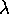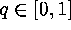Next: How to Catch ``Rare'' Up: The Scenario Machine: Operational Previous: Notes on Monte-Carlo Simulations

Dependence on the Scenario Parameters and Initial Distributions

Calculations of at least two runs with different values of a parameterare required in order to discover to what extent the results depend on the, if at all. However, statistical errors can significantly exceed a systematic trend caused by changing. To avoid this effect, we use a so-called method of differential experiment (Ermakov, 1985), when the same initial set of points (ZAMS-stars in our case) is used. In this case a systematic error acts similarly in all experiments and vanishes after the results have been subtracted to find the-trend.

In order to study how the final statistical distributions depend on the initial functions, the method of Green functions is used. Essentially, the interval of allowable values of the initial parameter (say, mass ratio) is divided into several parts, and we perform the experiment keeping the parameter uniformly distributed within each small interval. Then we can restore any distribution on this parameter (with a certain accuracy) by summing the results for each interval with the appropriate weight.

To study dependence on the star formation rate function, we can collect statistical data for different intervals of ages of the stars. Their subsequent convolution with appropriate weights allows us to obtain the results for an arbitrary dependence of the star formation rate on time.

Mike E. Prokhorov
Sat Feb 22 18:38:13 MSK 1997